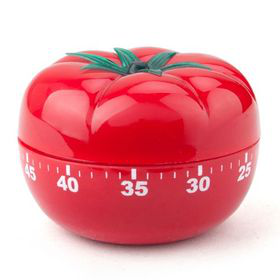Open in App
Not now

# Create a Pomodoro Using Python Tkinter

• Difficulty Level : Medium
• Last Updated : 22 Feb, 2022

In this article, we are going to see how to create a Pomodoro using Python Tkinter.

## Why Pomodoro?

Concentrating on studies or work is the most essential part of Time management. We always mess up time management when it comes to focusing on work. Fortunately, you can manage your time by focusing on your work and then taking a short break to relax. Pomodoro Technique is the more preferred technique to focus on the work for a specific time without any distraction. Pomodoro plays a major role in creating an effective time management system.

Firstly you need to take a task and work on it for straight 25 minutes without any distraction. Once the 25 minutes time period is done, take a short break of 5 minutes. In these 5 minutes, you can relax your brain by hearing music or short Podcasts. Repeat this process 4 to 5 times a day and you will see a drastic change.

## Create Pomodoro Timer Using Python Tkinter

Pomodoro starts with 25 minutes of work, you need to focus for 25 minutes. Once the 25 minutes, time period is done, using the Tkinter messagebox you can prompt the information. The same goes for the break period as well.

Now once we have imported the required libraries, the next thing is to create a GUI interference. Pomodoro means “Tomato” in Italian. In order to make this GUI look realistic, we will use a Tomato image as the background image. You can add the background image to the Tkinter application using the Canvas widget. Finally speaking about the final part of the project is to program the countdown timer.

We will use the time module to deal with the countdown timer. Since you are familiar with Pomodoro Technique now, first create a command function for 25 minutes of work. Initialize the minutes, seconds variable and store the total seconds of work and break time in a variable. Decrement the counter and update the GUI window every second. Once the count reaches zero, you should switch from work to break or vice versa. How will you get to know when the count is zero when you are focused on work? You can use the Tkinter messagebox here. Furthermore, you can even implement a playsound module and play small music when the count is 0.

Here is the trick for writing Timer Syntax in Tkinter:

Since the initial time is 25 minutes, first convert minutes into seconds.

## Python3

 `# timer = minutes*60` `timer ``=` `25``*``60` `#for work` `timer ``=` `5``*``60` `#for break`

Ok, now we have our timer ready we need to decrement it after every second. This can be implemented using time.sleep(1).

But the main issue might occur, in configuring minutes and seconds in the GUI. Since the timer is in seconds our decrement will also be in second i.e., 1500, 1499, 1498, and so on. To display the minute and second, we shall use the following formula:

```#for minutes: timer//60
#for seconds: timer%60```

For example, considering the 1366th second, we shall implement the above formula using divmod() function.

## Python3

 `# divmod syntax: divmod(x,y) => returns tuple (x//y,x%y)` `minute, second ``=` `divmod``(``1366``, ``60``)` `print``(minute)` `print``(second)`

Files Used:

sound.oggBelow is the implementation:

## Python3

 `import` `tkinter as tk` `from` `tkinter ``import` `messagebox` `from` `PIL ``import` `Image, ImageTk` `from` `playsound ``import` `playsound` `import` `time`   `class` `Pomodoro:` `    ``def` `__init__(``self``, root):` `        ``self``.root ``=` `root`   `    ``def` `work_break(``self``, timer):` `      `  `        ``# common block to display minutes` `        ``# and seconds on GUI` `        ``minutes, seconds ``=` `divmod``(timer, ``60``)` `        ``self``.``min``.``set``(f``"{minutes:02d}"``)` `        ``self``.sec.``set``(f``"{seconds:02d}"``)` `        ``self``.root.update()` `        ``time.sleep(``1``)`   `    ``def` `work(``self``):` `        ``timer ``=` `25``*``60` `        ``while` `timer >``=` `0``:` `            ``pomo.work_break(timer)` `            ``if` `timer ``=``=` `0``:` `              `  `                ``# once work is done play` `                ``# a sound and switch for break` `                ``playsound(``"sound.ogg"``)` `                ``messagebox.showinfo(` `                    ``"Good Job"``, "Take A Break, \` `                    ``nClick Break Button")` `            ``timer ``-``=` `1`   `    ``def` `break_(``self``):` `        ``timer ``=` `5``*``60` `        ``while` `timer >``=` `0``:` `            ``pomo.work_break(timer)` `            ``if` `timer ``=``=` `0``:` `              `  `                ``# once break is done,` `                ``# switch back to work` `                ``playsound(``"sound.ogg"``)` `                ``messagebox.showinfo(` `                    ``"Times Up"``, "Get Back To Work, \` `                    ``nClick Work Button")` `            ``timer ``-``=` `1`   `    ``def` `main(``self``):` `      `  `        ``# GUI window configuration` `        ``self``.root.geometry(``"450x455"``)` `        ``self``.root.resizable(``False``, ``False``)` `        ``self``.root.title(``"Pomodoro Timer"``)`   `        ``# label` `        ``self``.``min` `=` `tk.StringVar(``self``.root)` `        ``self``.``min``.``set``(``"25"``)` `        ``self``.sec ``=` `tk.StringVar(``self``.root)` `        ``self``.sec.``set``(``"00"``)`   `        ``self``.min_label ``=` `tk.Label(``self``.root,` `                                  ``textvariable``=``self``.``min``, font``=``(` `            ``"arial"``, ``22``, ``"bold"``), bg``=``"red"``, fg``=``'black'``)` `        ``self``.min_label.pack()`   `        ``self``.sec_label ``=` `tk.Label(``self``.root, ` `                                  ``textvariable``=``self``.sec, font``=``(` `            ``"arial"``, ``22``, ``"bold"``), bg``=``"black"``, fg``=``'white'``)` `        ``self``.sec_label.pack()`   `        ``# add background image for GUI using Canvas widget` `        ``canvas ``=` `tk.Canvas(``self``.root)` `        ``canvas.pack(expand``=``True``, fill``=``"both"``)` `        ``img ``=` `Image.``open``(``'pomodoro.jpg'``)` `        ``bg ``=` `ImageTk.PhotoImage(img)` `        ``canvas.create_image(``90``, ``10``, image``=``bg, anchor``=``"nw"``)`   `        ``# create three buttons with countdown function command` `        ``btn_work ``=` `tk.Button(``self``.root, text``=``"Start"``,` `                             ``bd``=``5``, command``=``self``.work,` `                             ``bg``=``"red"``, font``=``(` `            ``"arial"``, ``15``, ``"bold"``)).place(x``=``140``, y``=``380``)` `        ``btn_break ``=` `tk.Button(``self``.root, text``=``"Break"``,` `                              ``bd``=``5``, command``=``self``.break_,` `                              ``bg``=``"red"``, font``=``(` `            ``"arial"``, ``15``, ``"bold"``)).place(x``=``240``, y``=``380``)`   `        ``self``.root.mainloop()`     `if` `__name__ ``=``=` `'__main__'``:` `    ``pomo ``=` `Pomodoro(tk.Tk())` `    ``pomo.main()`

Output:

My Personal Notes arrow_drop_up
Related Articles